# Gaussian Beams

Excerpt from Field Guide to Lasers

Lasers often generate so-called Gaussian beams, where the transverse profile of the beam's electric field distribution can be described with a Gaussian function: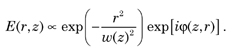Here, r is the distance from the beam axis, z is the coordinate along the propagation direction, w(z) is the so-called Gaussian beam radius, and φ(z,r) is a term describing the phase evolution along the beam as well as the curvature of the wavefronts: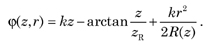Here, k = 2π / λ is the wave number, R(z) is the curvature of the wavefronts,
and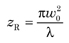is the Rayleigh length (or Rayleigh range) calculated from the beam radius w0
at the beam focus. The beam radius evolves according to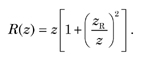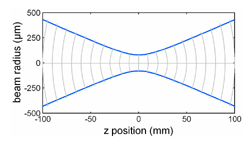The figure shows the evolution of the beam radius around the focus and also the curvature of the wavefronts, which is weak near and very far from the beam focus.

The beam intensity is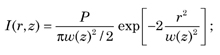for r = w, it reaches ≈13.5% of its value on the axis.

For z >> zR, the beam radius evolves in a nearly linear fashion, and the divergence angle is defined as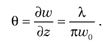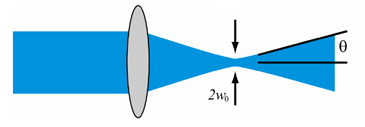The equation shows that the beam parameter product (BPP), defined as the product of beam waist radius w0 and the divergence angle, is λ / π and thus independent of w0. In fact, a Gaussian beam has the smallest possible (diffraction limited) BPP, which can be interpreted as the highest possible beam quality.

During propagation in a homogenous medium, a Gaussian beam stays Gaussian, only its parameters (beam radius, wavefront curvature radius, etc.) change. The same holds for propagation through thin lenses or for reflection at weakly curved mirrors. These properties give Gaussian beams an important role in optics, including the physics of optical resonators. Even for distinctly non-Gaussian beams, there is a generalization of Gaussian beam propagation (involving the so-called M2 factor) that can be widely used. However, Gaussian beam propagation breaks down for very strongly divergent beams (thus also for very tightly focused beams), as the analysis is based on the socalled paraxial approximation, which is then violated.

Citation:

R. Paschotta, Field Guide to Lasers, SPIE Press, Bellingham, WA (2008).